# Applying Scale Factors to Perimeter, Area, and Volume of Similar Figures

An error occurred trying to load this video.

Try refreshing the page, or contact customer support.

Coming up next: What Is a Number Line?

### You're on a roll. Keep up the good work!

Replay
Your next lesson will play in 10 seconds
• 0:05 Issues of Scale
• 0:37 What Is a Scale Factor?
• 2:04 Perimeter Examples
• 2:51 Area Examples
• 4:20 Volume Examples
• 6:41 Lesson Summary
Save Save

Want to watch this again later?

Timeline
Autoplay
Autoplay
Speed Speed

#### Recommended Lessons and Courses for You

Lesson Transcript
Instructor: Jeff Calareso

Jeff teaches high school English, math and other subjects. He has a master's degree in writing and literature.

How do shapes change sizes yet retain their proportions and similarities to other shapes? In this lesson, we'll look at what a scale factor is and how to apply it. We'll consider scale factors with regards to three different aspects of similar shapes: perimeter, area and volume.

## Issues of Scale

When I was a kid, I had all kinds of action figures - He-Man, Star Wars, Ghostbusters. I liked to play with them together, but that didn't always make sense. One set of action figures in particular, Thundercats, never fit. The Thundercats action figures were way bigger than all the other action figures. So, a Thundercat could never pilot a Star Wars speeder bike. And, my Han Solo figure was way too small for the Thundercats' vehicles. The scales just weren't the same. This is an example of why scale factors matter.

## What Is a Scale Factor?

A scale factor is simply a number that multiplies the dimensions of a shape. This can make a shape larger. Larger shapes will have a scale factor greater than one. So, if the scale factor is three, then the dimensions of the new shape will be three times larger than that of the original.

Let's say you own a doughnut shop, and you want a giant strawberry-frosted doughnut on top of your shop. You might use a scale factor of 25. Every tasty inch of a regular doughnut would be 25 inches on the model. So, a 5-inch doughnut would be 125 inches, or almost 10.5 feet tall.

A scale factor can also make a shape smaller. Smaller shapes will have a scale factor of less than one. You've probably seen this with Matchbox cars, which are often shrunk-down versions of real cars. This also works with dollhouses. For example, a model of a house may have a scale factor of 1/50. That means that 1 inch on the model is equal to 50 inches on the actual house. So, a 4-foot-tall dresser would be about 1 inch tall in the model.

If the scale factor is one, then the two shapes are the same, or congruent. The scale factor of the car below to the other car is one. That's not very interesting, so we don't usually talk about scale factors of one.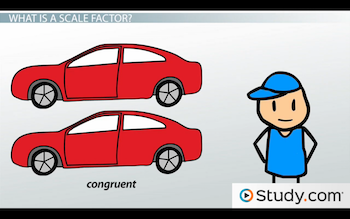If you're into variables, the equation for a scale factor can be written as y = Cx, where x is the original dimension, y is the new dimension, and C is the scale factor, or the amount by which the original is multiplied.

## Perimeter Examples

Let's talk about how scale factors influence shapes in geometry, starting with perimeter.

Here's a rectangle: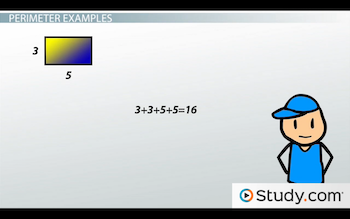Its width is 3 and its length is 5. What's the perimeter? 3 + 3 + 5 + 5, or 16. Now, let's apply a scale factor of 4 for the new rectangle. It will have dimensions that are 4 times that of the original. Instead of a width of 3, it will be 3 x 4, or 12. And, instead of a length of 5, it'll be 5 x 4, or 20. This new rectangle is similar to the original, which means it has the same shape, but not necessarily the same size.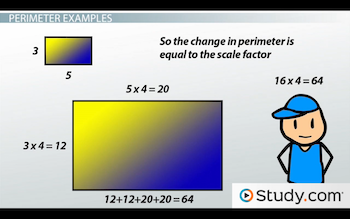What impact did this have on the perimeter? The new perimeter is 12 + 12 + 20 + 20, or 64. 16 to 64? That's 4 times the original. So, the change in perimeter is equal to the scale factor.

## Area Examples

Things are a little different with area. Let's look at those same two rectangles: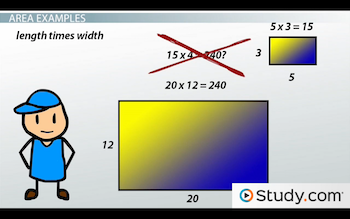What's the area of the first? The area of a rectangle is length times width, so it's 5 x 3, which is 15. What about the second one? 20 x 12, which is 240. Does 15 x 4 = 240? No. What is the relationship between 15 and 240? If you divide 240 by 15, you get 16. And, what was our scale factor? 4. 16 is 4^2. So, the change in area is equal to the scale factor squared.

Let's look at another example. Here's a triangle with a base of 5 and a height of 4: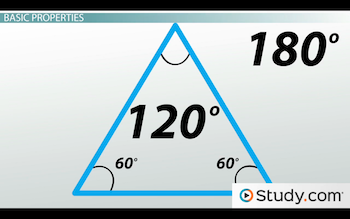The area of a triangle is ½ times base times height. So, this triangle's area is ½ x 5 x 4, which is 10. Let's make a new triangle using a scale factor of 3. This new triangle has a base of 15 and a height of 12. Its area is ½ x 15 x 12, or 90.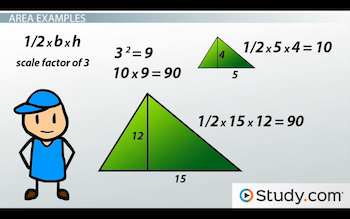Okay, remember that our scale factor was 3. And, 3^2? That's 9. So, the change in area should be 9 times the original. Does 10 x 9 = 90? Yes! So, it works.

When you're working with scale factors, square the scale factor to determine the area of the new figure. If you think about it, it makes sense why area would be the scale factor squared. Area involves two dimensions multiplied together. With scale factor, all you're really doing is multiplying the scale factor times itself.

To unlock this lesson you must be a Study.com Member.

### Register to view this lesson

Are you a student or a teacher?

#### See for yourself why 30 million people use Study.com

##### Become a Study.com member and start learning now.
Back
What teachers are saying about Study.com

### Earning College Credit

Did you know… We have over 200 college courses that prepare you to earn credit by exam that is accepted by over 1,500 colleges and universities. You can test out of the first two years of college and save thousands off your degree. Anyone can earn credit-by-exam regardless of age or education level.